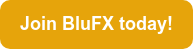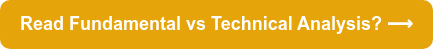Using technical analysis to predict changes in the market is crucial for a trader. Technical analysis essentially means looking at historical price movements in market data to spot patterns and seize valuable trading opportunities.

If you’re new to trading, it’s also important to understand fundamental analysis - you can read our What is Fundamental Analysis? blog here.# What is Technical Analysis in Forex?

It’s crucial for new traders to understand some technical analysis basics. Put simply, technical analysis is the study of currency price movement on the charts. It means making predictions based on previous data in a chart (see example below):It looks at the effects of market movements (unlike fundamental analysis, which looks at the causes of these movements) to get an idea of current trading conditions and potential price changes.

Technical analysis involves looking at a currency’s previous price to predict what might happen to that currency in the future - so you can buy or sell accordingly.

It’s important to note that the best forex technical analysts will understand that this type of analysis can be extremely subjective. For example, two traders looking at the same chart may develop different ideas about a currency’s value.

Technical analysis is used by traders to determine where the market may shift between bullish (an upward trend) and bearish (a downward trend).

You can see an example of a bullish trend below:And you can also see an example of a bearish trend below:## Methods of technical analysis

So, as we’ve established, methods of technical analysis involve using charts to spot trends and patterns in currency markets to find trading opportunities.

Technical analysis is largely based on the idea that history repeats itself and that patterns in stock markets repeat themselves due to market psychology (read more on Trading Psychology here) and patterns in emotions, like fear or excitement.

Traders will look at previous price points and then, on the basis that these levels will repeat themselves, make trades based on these patterns.

## Best forex technical analysis

So - what are the best forex technical analysis techniques?

You can use technical analysis to look at indicators of price movements, including: price trends; chart patterns; volume and momentum indicators; oscillators; moving averages and support and resistance levels.

These are some of things traders will look for when conducting technical analysis...

## Elliott waves

Elliott wave analysis is based on the Elliott Wave Theory, which is that psychology affects investment - the same patterns, upward or downward swings (or ‘waves’) recur over and over. It’s based on the idea that once you understand the structure of the waves, you can then predict where they will move next.

You can see an example of what an Elliott Wave would look like below:These patterns can be used as predictive indicators: an upwards trend is known as an impulse wave (as in the diagram above) while a downward trend is known as a corrective wave.

The Elliott Wave theory is based on a 5-3 pattern:

• Waves 1, 3 and 5 in the diagram above are impulse waves
• Waves 2 and 4 in the diagram above are corrective waves

Elliott Waves must also follow the rules below:

• Wave 2 never retraces more than 100% of wave 1
• Wave 3 cannot be the shortest of the three impulse waves, namely waves 1, 3 and 5
• Wave 4 does not overlap with the price territory of wave 1

So - how would you use Elliott Waves when trading?

As an example, if you see an impulse wave, you might go long (ie, buy in the hope that the currency will increase in value, and then sell later on). Or, if you see a corrective wave is imminent, you could go short (ie, sell with the idea of repurchasing later at a lower price).

## Pivot points

Pivot points are used by traders to determine where the market may shift between bullish (an upward trend) and bearish (a downward trend).

These pivot points are also used to find support and resistance levels, and are calculated using the open, high, low and close prices of the previous period (shown in candlestick charts - read more about these on our candlestick chart analysis blog).

So - how should you use pivot points when trading? Put simply, they are the points at which prices could change - so when a price reaches a support or resistance zone and then reverses (you can read more about identifying support or resistance levels on our blog, What Are Forex Trading Strategies?). You can see examples of support and resistance levels - and pivot points - below:In an uptrend, traders would look at the lower support points and wait for them to break. In a downtrend, you would wait for the higher resistance points to break.

So, if you see a price nearing the resistance level, you could sell the pair and place a stop just above the resistance. However, if you see a price nearing a support level, you could buy and put your stop just below the level.

## Fibonacci retracements

Fibonacci retracements, like pivot points, also make use of support and resistance levels. They are used as a predictive indicator to identify where a price might be in the future - and will give you a good indication of when to enter a trade.

The concept is that after a price begins a new trend direction, the price will retrace its steps back to a previous price level, before resuming the general direction of its trend.

What are the Fibonacci retracement levels?

The Fibonacci retracement levels are 76.4, 61.8, 38.2, and 23.6.

As you know, the following numbers - 0, 1, 1, 2, 3, 5, 8, 13, 21, 34, 55, 89, 144, and so on - are part of the Fibonacci sequence. The retracement levels above are linked to these numbers in the following ways:

• Each number is roughly 61.8% of the next number;
• Roughly 38.2% of the next number;
• And roughly 23.6% of the number after that
• Subtract 23.6 from 100, and the result is 76.4

These are your Fibonacci retracement levels: 76.4, 61.8, 38.2, and 23.6.

How do Fibonacci retracement levels work?

When a stock is trending very strongly in one direction, the idea is that the pullback will usually amount to one of the percentages included in the retracement levels: 23.6, 38.2, 61.8, or 76.4.

While trading, however, it’s important to remember that Fibonacci levels do not always pinpoint exact turning points. They provide an estimated entry area but not an exact entry point, so should be used with caution.

How should you use Fibonacci retracement levels during trading?

You should draw Fibonacci retracement levels - at 23.6, 38.2, 61.8, or 76.4 - on all significant price waves, noting where there is a cluster of Fibonacci levels to indicate where a price may retrace to.

The idea is to go long (buy) at a Fibonacci support level when the market is trending up (an impulse wave) - or to go short (sell) at a Fibonacci resistance level when the market is trending down (a corrective wave).

## What’s the difference between fundamental analysis and technical analysis?

Both fundamental and technical analysis are used to predict trends in the market, but there are differences between them. You can find out the difference between them on our blog, Forex Fundamental Analysis vs Technical Analysis.

Which is a long-term strategy and which is best for the short term? Find out the key differences between each type of analysis here…